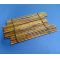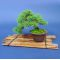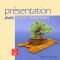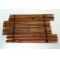##### The Japanese Bonsai specialist
Direct order Contact Help / Services Newsletter# Bamboo display shelves set of 2

› Bonsai tables and jita › Bonsai standsref. : 8569

15,00

Available quantity : 7Order

###### Description

Length: 30 cm.

Width: 9.5 cm.

Ø of the canes 3/5 mm.

Two mats put together: 30*19 cm.

Sold without the bonsai.

Orange-brown colour.

Set of two bamboo mats for presenting trees and plants at home or in exhibitions. The shelves enhance miniature bonsai and vivacious plants or kusamono. Put them side-by-side or use them separately.

#bonsai 4.6 #shelves 3.7 #bamboo 3.6 #display 2.7 #stands 2.6 #plants 2.6 #them 2.4 #side 2.4 #mats 2.4 #exhibitions 2.1

Formule
(( ROUND((CHAR_LENGTH(b.article_nom)-CHAR_LENGTH(REPLACE(b.article_nom, 'shelves', '')))/LENGTH('shelves')) + ROUND((CHAR_LENGTH(b.article_description)-CHAR_LENGTH(REPLACE(b.article_description, 'shelves', '')))/LENGTH('shelves')) ) * 3.7) + (( ROUND((CHAR_LENGTH(b.article_nom)-CHAR_LENGTH(REPLACE(b.article_nom, 'bamboo', '')))/LENGTH('bamboo')) + ROUND((CHAR_LENGTH(b.article_description)-CHAR_LENGTH(REPLACE(b.article_description, 'bamboo', '')))/LENGTH('bamboo')) ) * 3.6) + (( ROUND((CHAR_LENGTH(b.article_nom)-CHAR_LENGTH(REPLACE(b.article_nom, 'display', '')))/LENGTH('display')) + ROUND((CHAR_LENGTH(b.article_description)-CHAR_LENGTH(REPLACE(b.article_description, 'display', '')))/LENGTH('display')) ) * 2.7) + (( ROUND((CHAR_LENGTH(b.article_nom)-CHAR_LENGTH(REPLACE(b.article_nom, 'bonsai', '')))/LENGTH('bonsai')) + ROUND((CHAR_LENGTH(b.article_description)-CHAR_LENGTH(REPLACE(b.article_description, 'bonsai', '')))/LENGTH('bonsai')) ) * 2.6) + (( ROUND((CHAR_LENGTH(b.article_nom)-CHAR_LENGTH(REPLACE(b.article_nom, 'plants', '')))/LENGTH('plants')) + ROUND((CHAR_LENGTH(b.article_description)-CHAR_LENGTH(REPLACE(b.article_description, 'plants', '')))/LENGTH('plants')) ) * 2.6) + (( ROUND((CHAR_LENGTH(b.article_nom)-CHAR_LENGTH(REPLACE(b.article_nom, 'them', '')))/LENGTH('them')) + ROUND((CHAR_LENGTH(b.article_description)-CHAR_LENGTH(REPLACE(b.article_description, 'them', '')))/LENGTH('them')) ) * 2.4) + (( ROUND((CHAR_LENGTH(b.article_nom)-CHAR_LENGTH(REPLACE(b.article_nom, 'side', '')))/LENGTH('side')) + ROUND((CHAR_LENGTH(b.article_description)-CHAR_LENGTH(REPLACE(b.article_description, 'side', '')))/LENGTH('side')) ) * 2.4) + (( ROUND((CHAR_LENGTH(b.article_nom)-CHAR_LENGTH(REPLACE(b.article_nom, 'mats', '')))/LENGTH('mats')) + ROUND((CHAR_LENGTH(b.article_description)-CHAR_LENGTH(REPLACE(b.article_description, 'mats', '')))/LENGTH('mats')) ) * 2.4) + (( ROUND((CHAR_LENGTH(b.article_nom)-CHAR_LENGTH(REPLACE(b.article_nom, 'exhibitions', '')))/LENGTH('exhibitions')) + ROUND((CHAR_LENGTH(b.article_description)-CHAR_LENGTH(REPLACE(b.article_description, 'exhibitions', '')))/LENGTH('exhibitions')) ) * 2.1) + (( ROUND((CHAR_LENGTH(b.article_nom)-CHAR_LENGTH(REPLACE(b.article_nom, 'presenting', '')))/LENGTH('presenting')) + ROUND((CHAR_LENGTH(b.article_description)-CHAR_LENGTH(REPLACE(b.article_description, 'presenting', '')))/LENGTH('presenting')) ) * 2)

## Secure payment## Delivery

Our logistic partners :04 74 55 23 48
Pépinière MAILLOT-BONSAÏ
Le Bois Frazy
01990 RELEVANT - FRANCE
on appointment